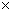Time: 3 Hours                                                                                                     Max. Marks: 100

NOTE: There are 9 Questions in all.

·      Question 1 is compulsory and carries 20 marks. Answer to Q. 1. must be written in the space provided for it in the answer book supplied and nowhere else.

·      Out of the remaining EIGHT Questions answer any FIVE Questions. Each question carries 16 marks.

·      Any required data not explicitly given, may be suitably assumed and stated.

Q.1       Choose the correct or best alternative in the following:                                         (2x10)

a.       Number of Terminals in a MOSFET are

(A)  Two                                             (B) Three

(C)    Four                                            (D) Five

b.      Which of the following material has the highest melting point

(A)    Copper.                                       (B)  Aluminium.

(C) Tungsten.                                      (D)  Gold.

c.   Bronze is an alloy of

(A)    Copper and Tin.                           (B)  Copper and Steel.

(C)  Copper and Mercury.                   (D)  Copper and Aluminium.

d.   A transistor has

(A)    One p-n junction.                        (B) Two p-n junction.

(C)  Four p-n junction.                        (D) Five p-n junction.

e.   Example of high resistivity material is

(A)     Nichrome                                     (B)  Silver

(C)  Gold                                            (D)  Copper

f.    Hard magnetic materials are used for making

(A)     Permanent magnets.                     (B)  Temporary magnets.

(C)  Conductors.                                 (D)  Insulator.

g.   Hall effect is associated with

(A)     Conductors.                                 (B)  Semiconductors.

(C)  Thermistors.                                 (D)  Solders.

h.   Addition of trivalent impurity to a semiconductor creates many

(A)   holes.                                           (B) free electrons.

(C) valance electrons.                          (D) bound electrons.

i.    Magnetic materials

(A)   provide path to magnetic flux.       (B)  are good insulators.

(C)  are semiconductors.                      (D)  None.

j.    Critical temperature above which ferromagnetic materials loose their magnetic property is called

(A)  Neutral temperature.                     (B)  Curie point.

(C)  Inversion temperature.                  (D) Normal temperature.

Answer any FIVE Questions out of EIGHT Questions.

Each question carries 16 marks.

Q.2     a.   Differentiate between diamagnetic, paramagnetic and ferromagnetic materials, give one example of each.                                                                (9)

b.   Draw B.H. curve for magnetic materials used in electric machines and explain

(i)   Hysteresis loop.                             (ii)  Permeability.                                     (7)

Q.3     a.   What are the important requirements of a good insulating material?                      (6)

b.   Write down the properties and uses of

(i)   porcelain                                       (ii)  glass

(iii) asbestos                                        (iv)  transformer oil                                (10)

Q.4           Discuss classification of conducting materials into low resistivity and high resistivity materials, give their properties and applications with examples.                                                        (16)

Q.5     a.   Explain temperature dependence of electrical resistivity and conductivity in conductors and semiconductors.                                                                                                                       (10)

b.   What are ferrities?  Give their properties and applications.                                   (6)

Q.6     a.   Explain the following:

(i)                  Polarization.

(ii)                Thermocouple.                                                                      (5+5)

b.   What is a pn junction?  Draw and explain VI characteristics of a pn junction diode.             (6)

Q.7           Write notes on any TWO :-

(i)                  Iron and silicon iron alloys.

(ii)                Electrical contact materials.

(iii)               Polymers and their applications.                                                         (8+8)

Q.8     a.   Discuss applications of dielectrics.                                                                    (10)

b.   Explain the effect of magnetic field on superconductivity.                                     (6)

Q.9           Write short notes on any FOUR:-

(i)                  Dielectric constant.

(ii)                Hall effect.

(iii)               Carbon and graphile.

(iv)              Ferroelectric materials.

(v)                Einstein relation (between diffusion constant and mobility)

(vi)              MOSFET.                                                                                      (44)